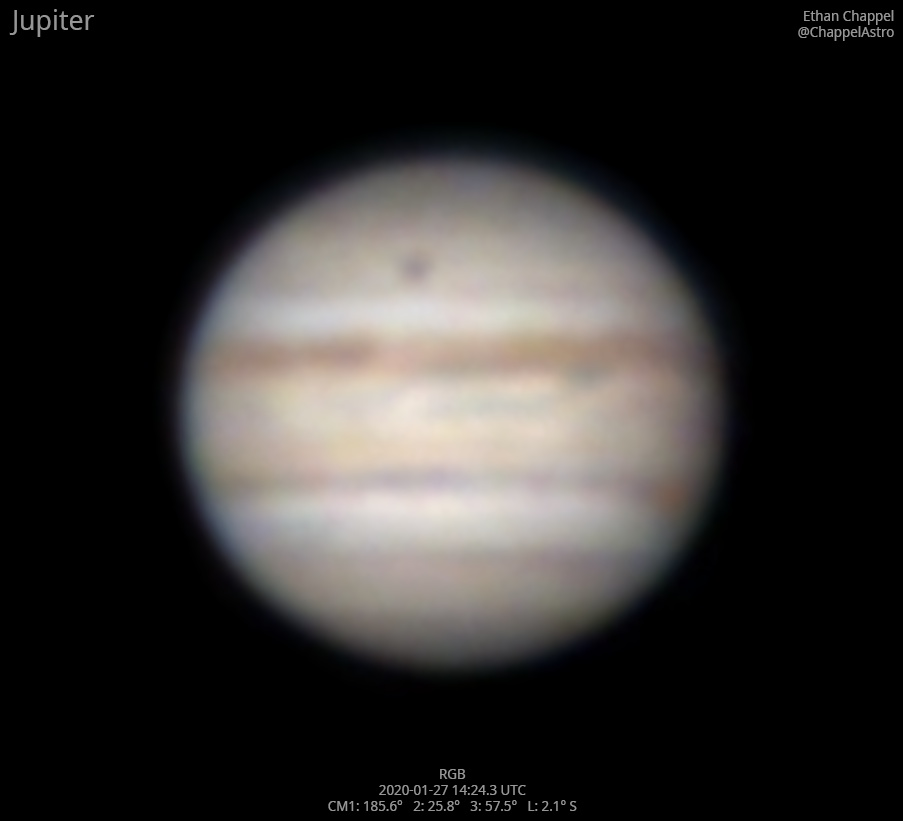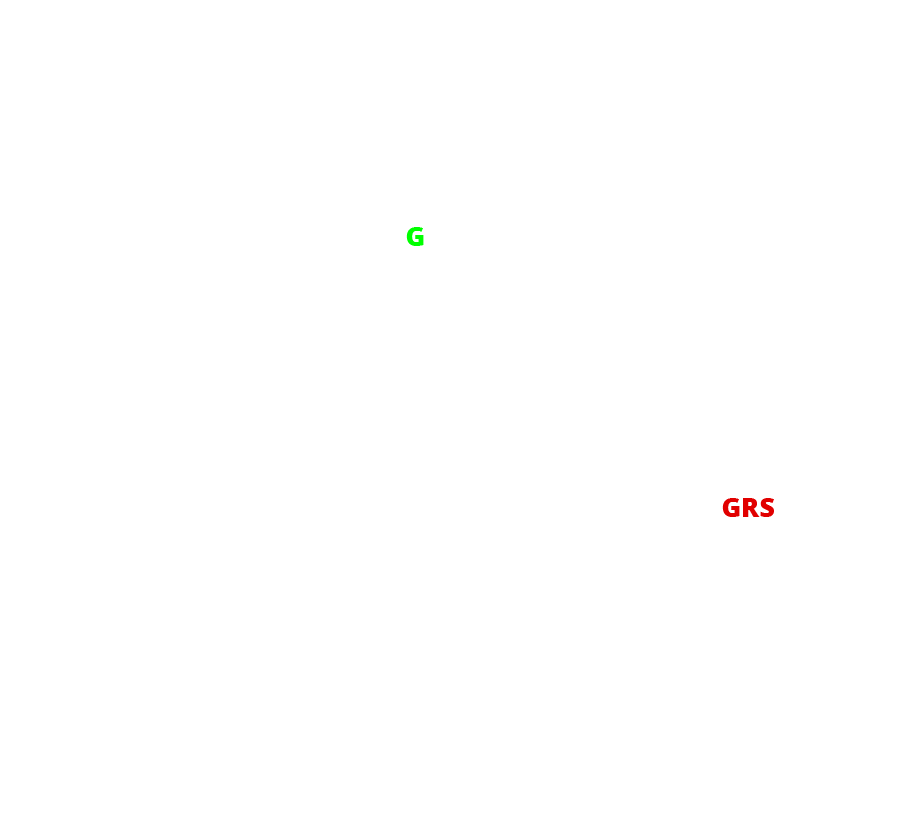# Jupiter 2020-01-27 14:24 UTC

CM1: 185.60°

CM2: 25.80°

CM3: 57.50°

CLat: +2.10°

Description

After finishing with Venus the previous evening, I refined the Advanced VX's alignment so the go-to was precise enough to center any target in an eyepiece. Doing so made Jupiter easy to find during the day. Started imaging about half an hour after sunrise. The blue channel was extremely challenging because of the low contrast between the planet and sky.

Notes:

• Ganymede is transiting over the North Temperate Zone.
• The Equatorial Zone is still orange. Hard to tell if it's still as intense as six months ago.
• The Great Red Spot is setting.

Logs
```FireCapture v2.6  Settings
------------------------------------
Observer=Ethan Chappel
Camera=ZWO ASI174MM
Filter=B
Profile=Jupiter
Diameter=32.28"
Magnitude=-1.86
CMI=188.9° CMII=29.1° CMIII=60.8°  (during mid of capture)
Filename=2020-01-27-1430_0-EC-B-Jup.ser
Date=2020_01_27
Start=14_28_33.686
Mid=14_30_03.687
End=14_31_33.689
Start(UT)=14_28_33.686
Mid(UT)=14_30_03.687
End(UT)=14_31_33.689
Duration=180.003s
Date_format=yyyy_MM_dd
Time_format=HH_mm_ss
LT=UT -6h
Frames captured=22364
File type=SER
Binning=no
Bit depth=8bit
Debayer=no
ROI=360x352
ROI(Offset)=0x0
FPS (avg.)=124
Shutter=8.000ms
Gain=205 (51%)
AutoExposure=off
Brightness=1 (off)
SoftwareGain=10 (off)
FPS=100 (off)
USBTraffic=80 (off)
HighSpeed=off
AutoHisto=75 (off)
Gamma=50 (off)
AutoGain=off
Histogramm(min)=131
Histogramm(max)=185
Histogramm=72%
Noise(avg.deviation)=4.22
AutoAlign=false
PreFilter=none
Limit=180 Seconds
Sensor temperature=26.1°C
FireCapture v2.6  Settings
------------------------------------
Observer=Ethan Chappel
Camera=ZWO ASI174MM
Filter=G
Profile=Jupiter
Diameter=32.28"
Magnitude=-1.86
CMI=185.8° CMII=26.0° CMIII=57.7°  (during mid of capture)
FocalLength=1400mm (F/5)
Resolution=0.87"
Filename=2020-01-27-1424_9-EC-G-Jup.ser
Date=2020_01_27
Start=14_23_26.401
Mid=14_24_56.408
End=14_26_26.415
Start(UT)=14_23_26.401
Mid(UT)=14_24_56.408
End(UT)=14_26_26.415
Duration=180.014s
Date_format=yyyy_MM_dd
Time_format=HH_mm_ss
LT=UT -6h
Frames captured=7765
File type=SER
Binning=no
Bit depth=8bit
Debayer=no
ROI=360x352
ROI(Offset)=0x0
FPS (avg.)=43
Shutter=23.14ms
Gain=104 (26%)
AutoExposure=off
Brightness=1 (off)
SoftwareGain=10 (off)
FPS=100 (off)
USBTraffic=80 (off)
HighSpeed=off
AutoHisto=75 (off)
Gamma=50 (off)
AutoGain=off
Histogramm(min)=123
Histogramm(max)=176
Histogramm=69%
Noise(avg.deviation)=2.34
AutoAlign=false
PreFilter=none
Limit=180 Seconds
Sensor temperature=25.6°C
FireCapture v2.6  Settings
------------------------------------
Observer=Ethan Chappel
Camera=ZWO ASI174MM
Filter=R
Profile=Jupiter
Diameter=32.28"
Magnitude=-1.86
CMI=181.6° CMII=21.8° CMIII=53.6°  (during mid of capture)
FocalLength=3250mm (F/11)
Resolution=0.37"
Filename=2020-01-27-1418_0-EC-R-Jup.ser
Date=2020_01_27
Start=14_16_35.899
Mid=14_18_05.906
End=14_19_35.914
Start(UT)=14_16_35.899
Mid(UT)=14_18_05.906
End(UT)=14_19_35.914
Duration=180.015s
Date_format=yyyy_MM_dd
Time_format=HH_mm_ss
LT=UT -6h
Frames captured=11965
File type=SER
Binning=no
Bit depth=8bit
Debayer=no
ROI=360x352
ROI(Offset)=0x0
FPS (avg.)=66
Shutter=15.00ms
Gain=220 (55%)
AutoExposure=off
Brightness=1 (off)
SoftwareGain=10 (off)
FPS=100 (off)
USBTraffic=80 (off)
HighSpeed=off
AutoHisto=75 (off)
Gamma=50 (off)
AutoGain=off
Histogramm(min)=112
Histogramm(max)=175
Histogramm=68%
Noise(avg.deviation)=4.33
AutoAlign=false
PreFilter=none
Limit=180 Seconds
Sensor temperature=24.6°C
```